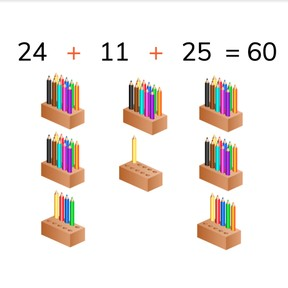No account needed.8,000 schools use Gynzy92,000 teachers use Gynzy1,600,000 students use Gynzy

## General

Students learn to add to 100 with three or more random addends. That means they are not specifically 10s, 5s, or immediately recognizable.

2.NBT.B.6
2.OA.A.1

## Relevance

It is important to be able to add to 100, so you can determine how many you have in total.

## Introduction

Students try to find a path through the numbers. They must add to exactly 100. Example: the red route starts with 0 and then 55 is added. Then 5 more, you now have 60. If I choose 15, I have 75, then I choose 5, 10, and 15 to reach the total of 100.

## Development

Discuss with students the importance of adding to 100. With the example problem on the board (36+23+34) ask students how they would solve the problem. Explain that you first look for the numbers that are easy to add together. In this case, 36+24 are easy because they add to a round number (60), and then you add the third addend. Also explain another method. In the second method you add all of the tens, and then all of the ones. Emphasize that students should choose the method that works best for them. Check that students are able to solve these problems by doing a few as a class.

Check that students are able to add to 100 with three or more random addends by asking the following questions:
- Why is it useful to be able to add to 100?
- Which method do you use to add three or more random numbers to 100?

## Guided practice

Students first practice adding with three and then four addends. They must then solve an addition problem in table form and finally a problem in story form.

## Closing

Check that students have understood by asking them how to solve an addition problem with three or more random addends to 100. Emphasize that every student is different and that your favourite method of solving might not be the same as your neighbor. One of each kind of addition problem is given (visual, abstract, story) to check student's understanding of adding with multiple random addends. Finally have students finish with a game. Ask a student to select a square. If there is a spider- you're out. If there isn't a spider, you get to go on. When four numbers have been uncovered, calculate the addition problem as a class.

## Teaching tips

Students who have difficulty adding to 100 with multiple random addends can practice by first skip counting in 10s to 100. They can use the number line for visual support. Making use of manipulatives like MAB blocks can also help. Start by solving problems with two random addends with a maximum of 100.

## Instruction materials

Optional: MAB blocks/ manipulatives, number line

### The online teaching platform for interactive whiteboards and displays in schools

• Save time building lessons

• Manage the classroom more efficiently

• Increase student engagement